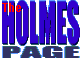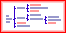THE EVOLUTION OF CODE BLOCKSCA-ClipperOpportunities Tips and Tricks Networking Internal Errors Source Code CA-VOBACK TO
CA-CLIPPER
PROGRAMMING

Introduction
Code blocks appeared for the first time in Clipper 5, but they did not just spring into existence -- there has been a steady progression towards them in the Clipper language.
Many concepts in Clipper are combined to implement code blocks. This document will attempt to show this in an easy, incremental manner.
First, A Reminder
Clipper is a language based on functions. Functions have return results. So, too, does the assignment operator `:=`.
 ``` X := 10 // Assign 10 to the variable called X. ? X // Print the value of X (which is 10).```
You could write:
 ` ? X := 10 // Assign 10 to X and print the result.`
Because of the order of precedence in Clipper, that is equivalent to:
 ` ? (X := 10)`
The expression `X := 10` is evaluated first, then its result (the value of `X`, which is now `10`) is passed to the `?` operator, which prints the value. Another way of thinking about it is "The value 10 goes into X and then keeps on going through to the print operator".
Another example is:
 ` store 0 to Z, Y, X`
Here is an alternate way of expressing the same idea:
 ` Z := Y := X := 0`
Again, because of the rules of precedence, it is evaluated like this:
 ` Z := ( Y := (X := 0) )`
Basically, from right to left (although Clipper normally goes from left to right). The value `0` is stored into `X`, and then it keeps on going into `Y`, and then into `Z`. In fact, it still keeps on going off the left-hand side of the page.
So if the code is changed to:
 ` ? Z := Y := X := 0`
the number `0` would appear on the screen. You could say that the value `0` "propagated through the expression", or "filtered through", or "bubbled up", or "kept on going".
Another Reminder
Clipper allows you to join several lines of code together on one line. For example, this code:
 ``` use CUSTOMER goto top```
could be written like this:
 ` use CUSTOMER ; goto top`
The semicolon tells Clipper that a new line of code is about to begin. This lets you put several related "logical" lines of code on the same "physical" line in your source code editor. Although you could write the entire program like this on one line (with semicolons), that wouldn't be recommended!Part 1: The Expression ListThe evolution of code blocks starts with the expression list.
In the samples below the `==>` symbol is meant to indicate what you would see on the screen.
Two Lines Of Code
 ``` ? X := 10 ==> 10 Y := 20```
Two Lines of Code On One, Using The Semicolon
This is the same code as above, just written on one line.
 ` ? X := 10 ; Y := 20 ==> 10`
Converted To An Expression List
Putting brackets around the code and replacing the semicolon with a comma makes it into an expression list:
 ` ? ( X := 10 , Y := 20 ) ==> 20`
An expression list is a "list of expressions". The value or return result of an expression list is the last thing in the list. It is a lot like a simple function, which returns the last thing.
So, this expression list evaluates `X := 10`, then evaluates `Y := 20`, then returns the last thing, which is then printed. After this code is complete, `X` will have a value of `10`, `Y` will be `20`, and `20` will appear on the screen.
 ``` ? X ==> 10 ? Y ==> 20```
Theoretically, any number of expressions can be combined into an expression list. In practice, the maximum is only about 500 symbols.
Debugging expression lists is hard because they are typed on one line of source code, which means that all component expressions will have the same line number associated with them. This can make it difficult to determine where the error occurred.
Where Would you Use An Expression List?
The primary purpose of the expression list is to group things together into one unit. Anywhere in Clipper a single expression can be used, you can put an expression list. Thus, you can make several things happen where only one normally would.
For example, here is an `if..else..endif` structure:
 ``` X := 10 ; Y := 20 if X > Y ? "X" Z := 1 else ? "Y" Z := -1 endif```
Here is the same concept, written using expression lists and the `iif()` function:
 ``` X := 10 ; Y := 20 iif( X > Y , ; ( qout("X"), Z := 1 ) , ; ( qout("Y"), Z := -1 ) )```
Notice that the `?` is gone, replaced by the `qout()` function. This is because expression lists can only contain expressions. But `?` is a command, not an expression. However, every command in Clipper has an equivalent function, so a search for `?` in the manual indicated that `qout()` was the function to use.
This kind of code would (hopefully) not be used in a real program, but it is shown here to give you an idea of the mechanics of expression lists.Part 2: From Expression List to Code BlockStarting With An Expression List
Here is the expression list again:
 ` ? ( X := 10 , Y := 20 ) ==> 20`
Which Is Similar To This Function
As mentioned earlier, an expression list is like a simple, one line function. Here is the function that is equivalent to the expression list above:
 ``` function F ( ) X := 10 Y := 20 return Y```
You would call the function like this:
 ` ? F() ==> 20`
With A Quick Stroke Of The Pen, Make A Code Block!
Here is where the magic happens. An expression list can be changed into a code block very easily.
 ``` ( X := 10 , Y := 20 ) // Expression List. {|| X := 10 , Y := 20 } // CODE BLOCK!```
Where The "{}" Things Come From
You may have seen the `{` `}` braces before. That's right -- arrays.
 ``` A := {10, 20, 30} // Pointer to DATA. B := {|| X := 10, Y := 20 } // Pointer to CODE.```
There's the secret: both data types are pointers to something. Arrays are pointers to data, while code blocks are pointers to code.
How Do You Print An Array Or A Code Block?
Since they are both pointers, they can not be printed out directly. Something more is needed. An array element is accessed by specifying and indexing number.
 ``` ? A // NO! Doesn't work. ? B // NO! Neither does this. ? A // YES! Works for an array, ? B // NO! Not for code block.```
Using an indexing number doesn't work for code blocks. Remember, code blocks are like expression lists, which are like simple functions. You can't execute a single line of a function, can you? It's all or nothing. So how do you "execute" a code block to get the result?
Use `eval()` To Evaluate A Code Block
A code block is evaluated (executed, run, triggered, fired, etc.) by using the `eval()` function.
 ` ? eval(B) ==> 20`
This function takes the code block (actually the pointer to the code block) and jumps to the beginning of the code, sort of like GOSUB in BASIC. Then it captures the return value of the code block ("the last thing in the list") and returns it.Part 3: Passing ParametersIf code blocks are like functions, then how are parameters passed to them?
First Look At The Function
Here is the sample function, modified to accept a parameter:
 ``` function F ( N ) // Declare the parameter. X := 10 Y := 20 + N // And use it. return Y```
Which Is Similar To This Code Block
Here is the code block, modified to accept a parameter:
 ` B := {| N | X := 10, Y := 20 + N}`
Notice the where the `N` appears, just like in the function.
Call The Function
 ` ? F(1) ==> 21`
Evaluate The Code Block
 ` ? eval(B, 1) ==> 21`Part 4: Using Code BlocksGiven the following array:
 ` A := {'GARY HALL', 'FRED SMITH', 'TIM JONES'}`
It can be sorted in ascending order with
 ` asort(A)`
The array now looks like:
 ` {'FRED SMITH', 'GARY HALL', 'TIM JONES'}`
It is sorted by first name.
The `asort()` function sorts in ascending order by default. That behaviour can be changed by providing a code block that sorts in descending order:
 ``` B := { | X, Y | X > Y } asort(A, B)```
The code block (according to the ascan() documentation) should be written to accept two parameters which are the two elements of the array to compare. Note that the code block does not know which two elements it is comparing -- ascan() selects the elements (perhaps using the QuickSort algorithm) and passes them to the code block.
The code block compares them and returns .T. if they are in the right order, or .F. if they are not. If the return value is .F., then ascan() swaps the two values in the array and moves on to the next pair of values. So, in the code block above, the comparison X > Y is .T. if the elements are in descending order, which means that the first value is greater than the second.
To sort on the last name, also in descending order:
 ` B := {|X,Y|substr(X,at(' ',X)+1) > substr(Y,at(' ',Y)+1)}`
Note that this code block looks for and compares the parts of the strings that immediately follow a space.
After using this code block, the array now contains:
 ` {'GARY HALL', 'TIM JONES', 'FRED SMITH'}`
Finally, to sort on a sub element (column) of an array use this code block:
 ` B := {|X, Y| X > Y}`Send comments about this site to Greg at gregh@ghservices.com All pages copyright © 1996-1999 GH Services™   Created 1996/10/01   Last updated 1999/09/30 All trademarks contained herein are the property of their respective owners